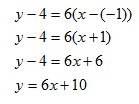Parallel Lines

Parallel lines are lines that do not intersect. Parallel lines have the same slope; using this property, an equation of a line parallel to a given line can be written.

Example 1 Find the equation of the line parallel to y = 6x - 9 that passes through point (-1, 4)

Step 1. Find the slope of the given line.

y = 6x - 9 is written in slope-intercept form and has a slope = 6

Step 2. Use the point-slope form to write the equation.y = 6x + 10 is the equation of the line passing through (-1, 4)

and parallel to the line y = 6x - 9

Listen